# Fraction calculator

The calculator performs basic and advanced operations with fractions, expressions with fractions combined with integers, decimals, and mixed numbers. It also shows detailed step-by-step information about the fraction calculation procedure. Solve problems with two, three, or more fractions and numbers in one expression.

## Result:

### 21/4 - 13/8 = 7/8 = 0.875

Spelled result in words is seven eighths.

### How do you solve fractions step by step?

1. Conversion a mixed number 2 1/4 to a improper fraction: 2 1/4 = 2 1/4 = 2 · 4 + 1/4 = 8 + 1/4 = 9/4

To find new numerator:
a) Multiply the whole number 2 by the denominator 4. Whole number 2 equally 2 * 4/4 = 8/4
b) Add the answer from previous step 8 to the numerator 1. New numerator is 8 + 1 = 9
c) Write a previous answer (new numerator 9) over the denominator 4.

Two and one quarter is nine quarters
2. Conversion a mixed number 1 3/8 to a improper fraction: 1 3/8 = 1 3/8 = 1 · 8 + 3/8 = 8 + 3/8 = 11/8

To find new numerator:
a) Multiply the whole number 1 by the denominator 8. Whole number 1 equally 1 * 8/8 = 8/8
b) Add the answer from previous step 8 to the numerator 3. New numerator is 8 + 3 = 11
c) Write a previous answer (new numerator 11) over the denominator 8.

One and three eighths is eleven eighths
3. Subtract: 9/4 - 11/8 = 9 · 2/4 · 2 - 11/8 = 18/8 - 11/8 = 18 - 11/8 = 7/8
For adding, subtracting, and comparing fractions, it is suitable to adjust both fractions to a common (equal, identical) denominator. The common denominator you can calculate as the least common multiple of both denominators - LCM(4, 8) = 8. In practice, it is enough to find the common denominator (not necessarily the lowest) by multiplying the denominators: 4 × 8 = 32. In the next intermediate step, the fraction result cannot be further simplified by canceling.
In words - nine quarters minus eleven eighths = seven eighths.

#### Rules for expressions with fractions:

Fractions - use the slash “/” between the numerator and denominator, i.e., for five-hundredths, enter 5/100. If you are using mixed numbers, be sure to leave a single space between the whole and fraction part.
The slash separates the numerator (number above a fraction line) and denominator (number below).

Mixed numerals (mixed fractions or mixed numbers) write as non-zero integer separated by one space and fraction i.e., 1 2/3 (having the same sign). An example of a negative mixed fraction: -5 1/2.
Because slash is both signs for fraction line and division, we recommended use colon (:) as the operator of division fractions i.e., 1/2 : 3.

Decimals (decimal numbers) enter with a decimal point . and they are automatically converted to fractions - i.e. 1.45.

The colon : and slash / is the symbol of division. Can be used to divide mixed numbers 1 2/3 : 4 3/8 or can be used for write complex fractions i.e. 1/2 : 1/3.
An asterisk * or × is the symbol for multiplication.
Plus + is addition, minus sign - is subtraction and ()[] is mathematical parentheses.
The exponentiation/power symbol is ^ - for example: (7/8-4/5)^2 = (7/8-4/5)2

#### Examples:

subtracting fractions: 2/3 - 1/2
multiplying fractions: 7/8 * 3/9
dividing Fractions: 1/2 : 3/4
exponentiation of fraction: 3/5^3
fractional exponents: 16 ^ 1/2
adding fractions and mixed numbers: 8/5 + 6 2/7
dividing integer and fraction: 5 ÷ 1/2
complex fractions: 5/8 : 2 2/3
decimal to fraction: 0.625
Fraction to Decimal: 1/4
Fraction to Percent: 1/8 %
comparing fractions: 1/4 2/3
multiplying a fraction by a whole number: 6 * 3/4
square root of a fraction: sqrt(1/16)
reducing or simplifying the fraction (simplification) - dividing the numerator and denominator of a fraction by the same non-zero number - equivalent fraction: 4/22
expression with brackets: 1/3 * (1/2 - 3 3/8)
compound fraction: 3/4 of 5/7
fractions multiple: 2/3 of 3/5
divide to find the quotient: 3/5 ÷ 2/3

The calculator follows well-known rules for order of operations. The most common mnemonics for remembering this order of operations are:
PEMDAS - Parentheses, Exponents, Multiplication, Division, Addition, Subtraction.
BEDMAS - Brackets, Exponents, Division, Multiplication, Addition, Subtraction
BODMAS - Brackets, Of or Order, Division, Multiplication, Addition, Subtraction.
GEMDAS - Grouping Symbols - brackets (){}, Exponents, Multiplication, Division, Addition, Subtraction.
Be careful, always do multiplication and division before addition and subtraction. Some operators (+ and -) and (* and /) has the same priority and then must evaluate from left to right.

## Fractions in word problems:

• Math testObelix filled a mathematical test in which he answered 25 questions. For every correct answer, he received 5 points, for each bad answer he had 3 points deducted. Obelix gained 36% of all points in the test. How many questions did he solve correctly?
• Package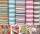The package was 23 meters of textile. The first day sold 12.3 meters. How many meters of textile remained in the package?
• IssacIssac eats 1/6 of the pizza. Maya then eats 3/5 of the remaining pizza. What fraction of the original pizza is left?
• Of the 2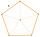Of the shapes,1/6 are triangles and 5/12 are pentagons. What fraction of the shapes are either triangles or pentagons?
• Rita hasRita has 3/4 m of Ifugao cloth. She used 2/3 m for placement. What part of Ifugao cloth was left?
• CerealsAri and Joey share a 30-ounce box of cereal. By the end of the week, Ari has eaten 3/10 of the box, and Joey has eaten 3/5 of the box of cereal. How many ounces are left in the box?
• Bathroom 4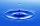Dolor puts 3 1/2 pails of water into a water container in the bathroom every day. Her daughter, Lei, uses 2 1/4 pails of water every day when taking a bath. If the water container had 5 5/8 pails of water at the start, how much water is left in it after 5
• PediatricianPediatrician this month of 20 working days takes 8 days holidays. What is the probability that on Monday it will be at work?
• The Mayflower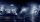The Mayflower traveled for 66 days on the trip from England to America. The weather was storming for many days of their trip. If one and a half of the days at Sea where Sunny with good weather, 1/6 of the days were sunny but very windy and the other days
• Fraction expressionWhich expression is equivalent to : minus 9 minus left parenthesis minus 4 start fraction 1 divided by 3 end fraction right parenthesis
• Pizza fractionsAnn ate a third of a pizza and then another quater. Total part of pizza eaten by Ann and how much pizza is left?
• Two pizzasJacobs mom bought two whole pizzas. He ate 2/10 of the pizza and his dad ate 1 1/5. How much is left.
• Visit to grandmother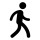Robert's family traveled 5/8 of the distance to his grandmother’s house on Saturday. They traveled 1/3 of the remaining distance on Sunday. What fraction of the total distance to his grandmother’s house was traveled on Sunday?